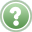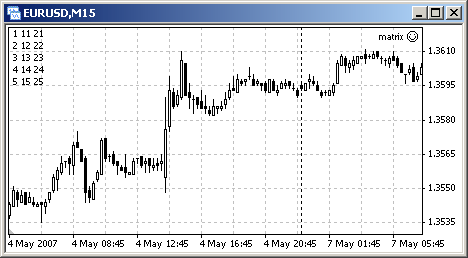English

MQL4 BookStandard FunctionsArrays and Timeseries

# Arrays and Timeseries

It is very important to keep in mind that the sequence of any single-type elements is always numbered starting from zero in MQL4.

It was mentioned before that you shouldn't confuse the value of the array element index with the number of elements in the array (see Arrays). For example, if the array is declared:

`  int Erray_OHL;         // Array declaration`

then it means that a one-dimensional array named Erray_OHL contains three elements. Indexing of the elements start with zero, i.e. the first of three elements has the 0 index (Erray_OHL), the second one - the 1 index (Erray_OHL), and the third one - the 2 index (Erray_OHL). In such a way, the maximum index value is less than the number of the elements in array by one. In this case, the array is one-dimensional, i.e. we can say about the amount of elements in the first dimension: the maximum index number is 2, because the number of the elements in the array is 3.

The same thing can be said about the numeration of the dimensions in the array. For example, if an array is declared the following way:

`  int Erray_OHL;      // Array declaration`

it means that the array has two dimensions. The first dimension specifies the number of rows (3 in this example), and the second one specifies the number of elements in the row (or the number of columns, 8 in this example). The dimension itself is numerated too. The first dimension has the 0 number, and the second one - the 1 number. The numbers of dimensions are used in the ArrayRange() function, for example.

## ArrayRange() Function

` int ArrayRange(object array[], int range_index)`

The function returns the number of elements in the specified dimension of the array.

The use of ArrayRange() function can be demonstrated with the solution of the following problem:Problem 38. The Mas_1 array contains the values of the 3x5 matrix. Get the values of the Mas_2 array that contains the elements whose values are equal to the values of the transposable matrix. Use arbitrary values of the elements.

Let's work out some values of the elements and represent the initial and the desired matrices that the Mas_1 and Mas_2 arrays contain respectively:

 Indexes 0 1 2 3 4 0 1 2 3 4 5 1 11 12 13 14 15 2 21 22 23 24 25
 Indexes 0 1 2 0 1 11 21 1 2 12 22 2 3 13 23 3 4 14 24 4 5 15 25
Initial matrix, Mas_1 array. Transposable matrix, Mas_2 array.

Fig. 151. Initial and Transposable Matrices.

In this case, the problem resolves itself to rewriting the values of the first matrix to the second one according to the rules of matrix transposition, namely rewrite the elements values of the first matrix columns to the rows of the desired matrix. The solution of matrix transposition problem is represented in the matrix.mq4 expert:

`//--------------------------------------------------------------------// matrix.mq4// The code should be used for educational purpose only.//--------------------------------------------------------------- 1 --int start()                            // Special function start()  {   int Mas_1={1,2,3,4,5,  11,12,13,14,15,  21,22,23,24,25};   int Mas_2;   int R0= ArrayRange( Mas_1, 0);      // Number of elements in first dim.   int R1= ArrayRange( Mas_1, 1);      // Number of elements in second dim.    for(int i=0; i`

Two arrays are opened in the start() function of the expert. The Mas_1 array has 3 rows containing 5 elements each and the MAS_2 array has 5 rows containing 3 elements each. The rewriting of the values itself is performed in the following entry:

`         Mas_2[[j][i] = Mas_1[i][j];    // Matrix transposition`

In order to calculate the runtime environment (the number of iterations) of two embedded cycle operators, you should know the values of the elements of each array. In this example the constant values 3 and 5 could be used. However, this way of program designing is incorrect. In general, a program can contain a huge code wherein the calling to the same values is performed in many parts of it. A program must be designed so that the modifications could be made in one place, if necessary, and in all the other necessary parts they would be calculated. In this case, only the entries that open and initialize the arrays should be modified if it is necessary to change the size of the arrays, so there is no need to modify the code in the other parts.

To determine the number of elements of the first and second dimensions of the Mas_1 array the following calculations are performed:

`   int R0 =  ArrayRange( Mas_1, 0);    // Number of elements in first dim.   int R1 =  ArrayRange( Mas_1, 1);    // Number of elements in second dim.`

Note that the 0 value is used for the first dimension and the 1 value is used for the second one. The calculated values of the R0 and R1 variables are used to determine the number of iterations in the "for" cycles.

The received values of the Mas_2 array elements are displayed on the screen using the Comment() function.Fig. 152.Result of matrix.mq4 operation.

## Functions for Working with Arrays

Functions Short Description
ArrayBsearch It returns the index of the first found element in the first dimension of array. If the element with the specified value ia absent then the function will return the index of the nearest (by value) element.
ArrayCopy It copies one array to another. The arrays must have the same type. The arrays of the double[], int[], datetime[], color[], and bool[] types can be copied as the arrays of the same type. Returns the number of copied elements.
ArrayCopyRates It copies the bar data to the two-dimensional array of the RateInfo[] kind and returns the number of copied bars. Otherwise it returns -1, if the operation fails.
ArrayCopySeries It copies a timeseries array to the user-defined array and returns the number of copied elements.
ArrayDimension It returns rank of a multy-dimensional array.
ArrayGetAsSeries It returns TRUE if the array is arranged as a timeseries (elements of the array are indexed from the last element to the first one), otherwise returns FALSE.
ArrayInitialize It sets a single value to all elements of the array. Returns the number of initialized elements.
ArrayIsSeries It returns TRUE if the checked array is a timeseries (Time[], Open[],Close[],High[],Low[] of Volume[]), otherwise returns FALSE.
ArrayMaximum It searches an elemnet with the maximum value. The function returns the location of the maximum element in the array.
ArrayMinimum It searches an element with the minimum value. The function returns the location of the minimum element in the array.
ArrayRange It returns the number of elements in the specified dimension of the array. The size of the dimension is greater than the biggest index by 1, because the indexes are starting with zero.
ArrayResize It sets a new size of the first dimension of the array. Returns the number of all elements that array contains after its rank has been changed if the function ran successfully, otherwise returns -1 and the size of the array is not changed.
ArraySetAsSeries It sets the direction of indexing in the array.
ArraySize It returns the number of elements in an array.
ArraySort It sorts numeric arrays by their first dimension. The timeseries arrays cannot be sorted.

## Functions to Access Timeseries

Functions Summary Info
iBars It returns the number of bars of the specified chart.
iBarShift It searches a bar by time. The function returns the shift of bar that has the specified time. If the bar for the specified time is absent ("hole" in the history) then the function returns -1 depending on the exact parameter or the shift of the nearest bar.
iClose The function returns the close price of the bar specified with the shift parameter from the corresponding chart (symbol, timeframe). It returns 0, if an error occurs.
iHigh It returns the maximum price value of the bar specified with the shift parameter from the corresponding chart (symbol, timeframe). It returns 0, if an error occurs.
iHighest It returns the index of the maximum found value (shift relatively to the current bar).
iLow It returns the minimum price value of the bar specified with the shift parameter from the corresponding chart (symbol, timeframe). It returns 0, if an error occurs.
iLowest It returns the index of the minimum found value (shift relatively to the current bar).
iOpen It returns the open price value of the bar specified with the shift parameter from the corresponding chart (symbol, timeframe). It returns 0, if an error occurs.
iTime It returns opening time of the bar specified with the shift parameter from the corresponding chart (symbol, timeframe). It returns 0, if an error occurs.
iVolume It returns tick volume value of the bar specified with the shift parameter from the corresponding chart (symbol, timeframe). It returns 0, if an error occurs.

To get the detailed information about these and other functions, please refer to the Documentation at MQL4.community, at MetaQuotes Ltd. website or at the "Help" section of MetaEditor.File OperationsMathematical Functions